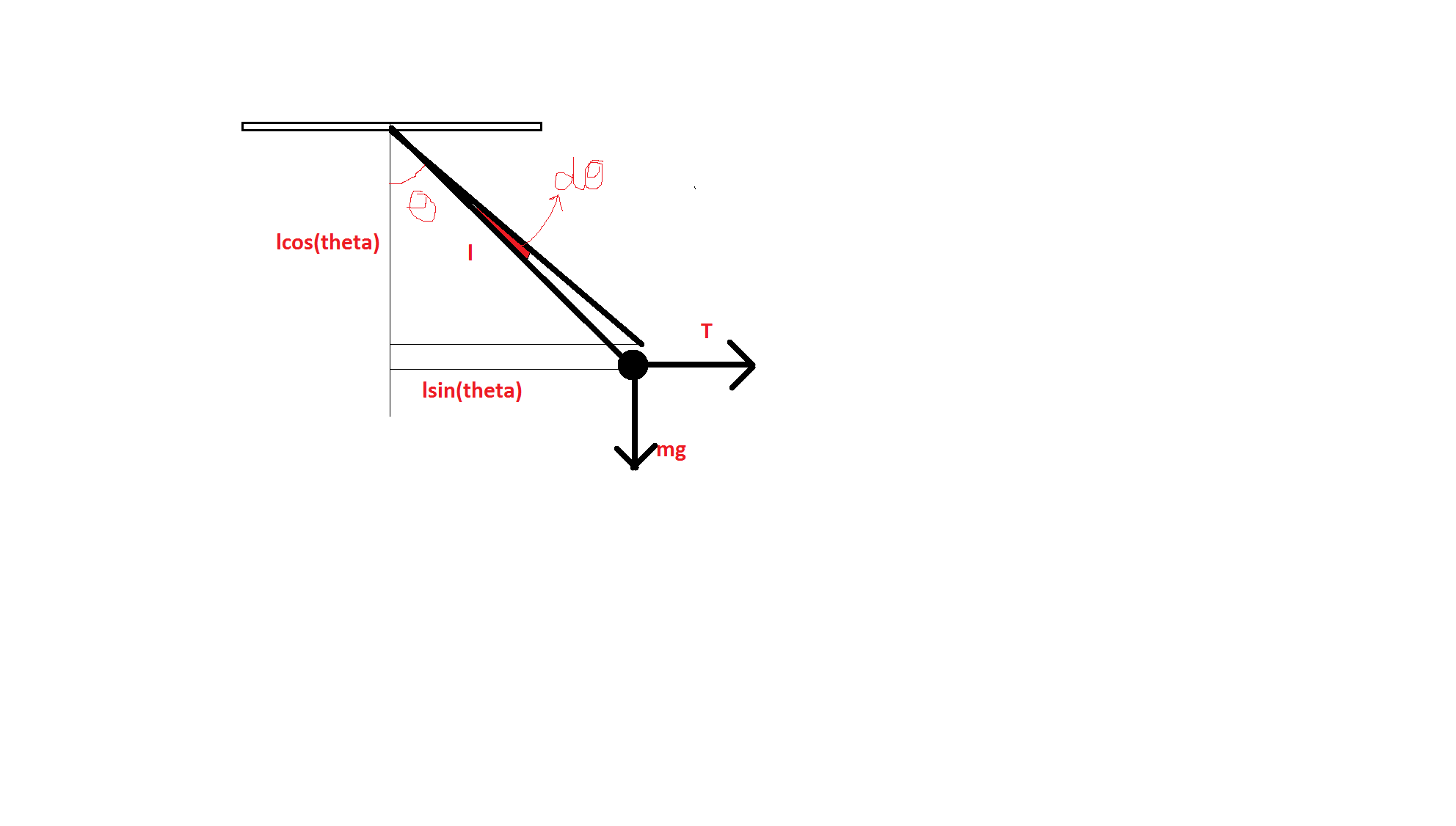# Virtual Work Method

Hello Physicists!

I am studying Newton's Laws of Motion, and am working out some problems on Pulleys and Constrained motion. I have heard of a technique called the Virtual Work Method, also called the T dot Trick or the Tension Trick. I would like to learn about it, to make my problem solving effective. So please write about it in this note and attach an example problem. I'll be highly grateful.

Thanks.

SwapnilNote by Swapnil Das
5 years, 3 months ago

This discussion board is a place to discuss our Daily Challenges and the math and science related to those challenges. Explanations are more than just a solution — they should explain the steps and thinking strategies that you used to obtain the solution. Comments should further the discussion of math and science.

When posting on Brilliant:

• Use the emojis to react to an explanation, whether you're congratulating a job well done , or just really confused .
• Ask specific questions about the challenge or the steps in somebody's explanation. Well-posed questions can add a lot to the discussion, but posting "I don't understand!" doesn't help anyone.
• Try to contribute something new to the discussion, whether it is an extension, generalization or other idea related to the challenge.

MarkdownAppears as
*italics* or _italics_ italics
**bold** or __bold__ bold
- bulleted- list
• bulleted
• list
1. numbered2. list
1. numbered
2. list
Note: you must add a full line of space before and after lists for them to show up correctly
paragraph 1paragraph 2

paragraph 1

paragraph 2

[example link](https://brilliant.org)example link
> This is a quote
This is a quote
    # I indented these lines
# 4 spaces, and now they show
# up as a code block.

print "hello world"
# I indented these lines
# 4 spaces, and now they show
# up as a code block.

print "hello world"
MathAppears as
Remember to wrap math in $$ ... $$ or $ ... $ to ensure proper formatting.
2 \times 3 $2 \times 3$
2^{34} $2^{34}$
a_{i-1} $a_{i-1}$
\frac{2}{3} $\frac{2}{3}$
\sqrt{2} $\sqrt{2}$
\sum_{i=1}^3 $\sum_{i=1}^3$
\sin \theta $\sin \theta$
\boxed{123} $\boxed{123}$

Sort by:

The crux of the Virtual Work Method is that the sum of work done by all internal forces of a system is zero!. So to explain this in the context of pulley block system I'll consider bodies $B_1,B_2,B_3\dots B_N$ which, due to the strings they are attached to, are experiencing a tension of $\vec{T_1},\vec{T_2}\dots \vec{T_N}$. Also, consider that in a certain time $t$ these blocks get displaced by $\vec{S_1},\vec{S_2}\dots \vec{S_N}$. Since the total work done by all internal forces is zero, we can write:

$\boxed\sum_{i=0}^{N} \vec{T_{i}}.\vec{S_{i}} = 0$...$(1)$

Differentiating the above equation twice we get:

$\boxed\sum_{i=0}^{N} \vec{T_{i}}.\vec{a_{i}} = 0$ (Here $\vec{a_i}$ denotes the accelleration of the body $B_i$)

This method is basically used to find the relationship between the accelerations of the various moving bodies of the system.

- 5 years, 2 months ago

This one helped me ;)

- 5 years, 2 months ago

virtual work is a really a god trick when you get fed up with constrains writing static equilibrium condition . virtual work principle state that when a body in static equilibrium is given a virtual displacement dx then net work done is 0.

- 5 years, 3 months ago

it sounds too boring but its going to be interesting i found it to be useful. try this

a mass tied with a string in vertical plane with horizontal force acting find it if it is at angle theta with the vertical using virtual work method. (it s too easy)

- 5 years, 3 months agojust give a displacement d(theta) and let net work done =0

mg(-lsin(theta)d(theta)+F(lcos(theta)d(theta)=0

F=mgtan(theta)

{note-this may appear long this time but its time saving trick }

- 5 years, 3 months ago

How is virtual tension work method for wedge pulley constraints different from pulleys?

- 4 years, 7 months ago

- 5 years, 3 months ago

It basically says $\displaystyle \sum \vec{T} \cdot \vec{a} = 0$ where $T$ is the tension of the string and $a$ is the acceleration of bodies in contact with it.

- 5 years, 3 months ago

Could you add one example? Thanks.

- 5 years, 3 months ago

Also can anybody please explain in brief about instantaneous axis of rotation.

- 5 years, 3 months ago

It is the point about which the body appers to be in purely rotational motion (no translatory motion).

- 5 years, 3 months ago

How to apply this concept in problem solving?

- 5 years, 3 months ago

You can figure out the ratios of veloties of certain points if you know their distance from the ICR and the angular velocity. Plus, you can calculate kinetic energy by knowing the moment of intertia about that point (you don't need to calculate translational kinetic energy).

- 5 years, 3 months ago

@Swapnil Das I think this'll help: https://nptel.ac.in/courses/Webcourse-contents/IIT-KANPUR/enggmechanics/ui/Coursehome_10.htm

- 2 years, 11 months ago